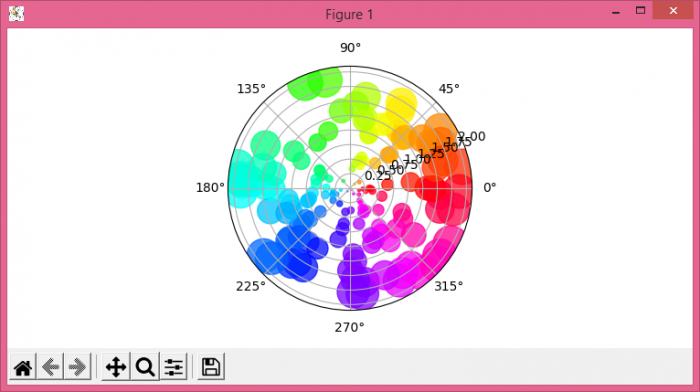# Plot scatter points on polar axis in Matplotlib

To plot scatter points on polar axis in Matplotlib, we can take the following steps −

• Set the figure size and adjust the padding between and around the subplots.
• Initialize a variable, N, for number of sample data.
• Get r, theta, area and color data using numpy
• Create a new figure or activate an existing figure.
• Plot theta, r, colors and area, using scatter() method.
• To display the figure, use show() method.

## Example

import numpy as np
import matplotlib.pyplot as plt

plt.rcParams["figure.figsize"] = [7.50, 3.50]
plt.rcParams["figure.autolayout"] = True

N = 150
r = 2 * np.random.rand(N)
theta = 2 * np.pi * np.random.rand(N)
area = 200 * r**2
colors = theta

fig = plt.figure()
plt.show()